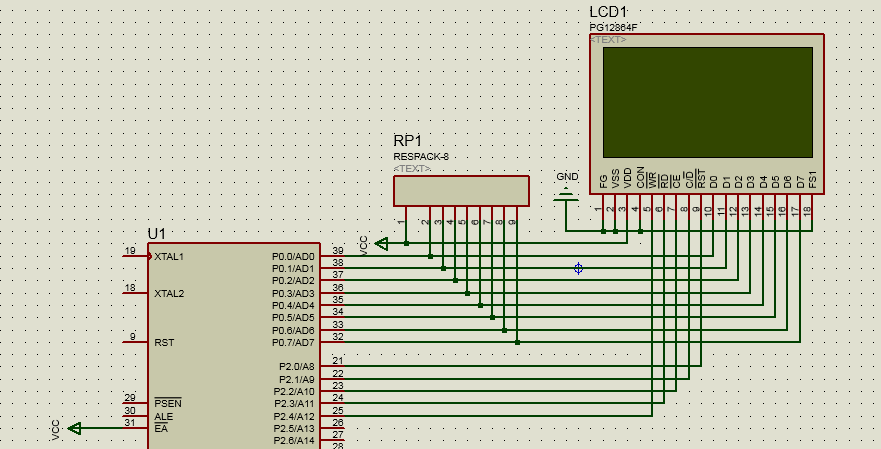• 首页
• 论坛
• 版块

• 问答
• 活动
• 下载
• 学院
• 发现

• 工作
• 升级VIP

[问答]

求大神分享基于proteus的51单片机仿真LCD1286445
2021-9-28 08:51:40评论淘帖 邀请回答

相关推荐

< >
1个回答
 1.0 电路图2.0 程序           uchar num[] = "0123456789";           sbit RS = P2^2; sbit RW = P2^1; sbit E = P2^0;        void delay(uint x) {         while(x--);        } /***向LCD写一个命令**/ void write_command(uchar command) {         RW = 0;         RS = 0;         P0 = command;         E = 1;         delay(100);//等待接收，忽略读状态操作         E = 0;         RW = 1; } /***向LCD写一个数据**/ void write_data(uchar date) {         RW = 0;         RS = 1;         P0 = date;         E = 1;         delay(100);//等待接收         E = 0;         RW = 1; } /**初始化**/ void FLCD1602_init(void) {         write_command(0x38);//两行，每行16个字符，每个字符是5*7点阵         write_command(0x0f);//光标显示并闪烁         //write_command(0x0c);//光标不显示         write_command(0x06);//光标随字符右移 }   /**清屏**/ void FLCD1602_clear() {         write_command(0x01);         write_command(0x02); } /**向LCD写字符串**/ void display_string(uchar *p) {         while(*p)//字符非空         {                 write_data(*p);//写字符                 p++;             //数据指针++         } } /**向LCD写数字变量**/ void display_num(uchar x) {         write_data(num[x / 10]);         write_data(num[x % 10]); } /**定位**/ void gotoxy(uchar y,uchar x) {         if(y == 1)         write_command(0x40 + x);         else if(y == 2)         write_command(0x80 + 0x40 + x); }

创建小组步骤

•最新主题
•推荐主题
•热门主题
•我的帖子
-

-

-

-

-

-

-

-

-

-

-

-